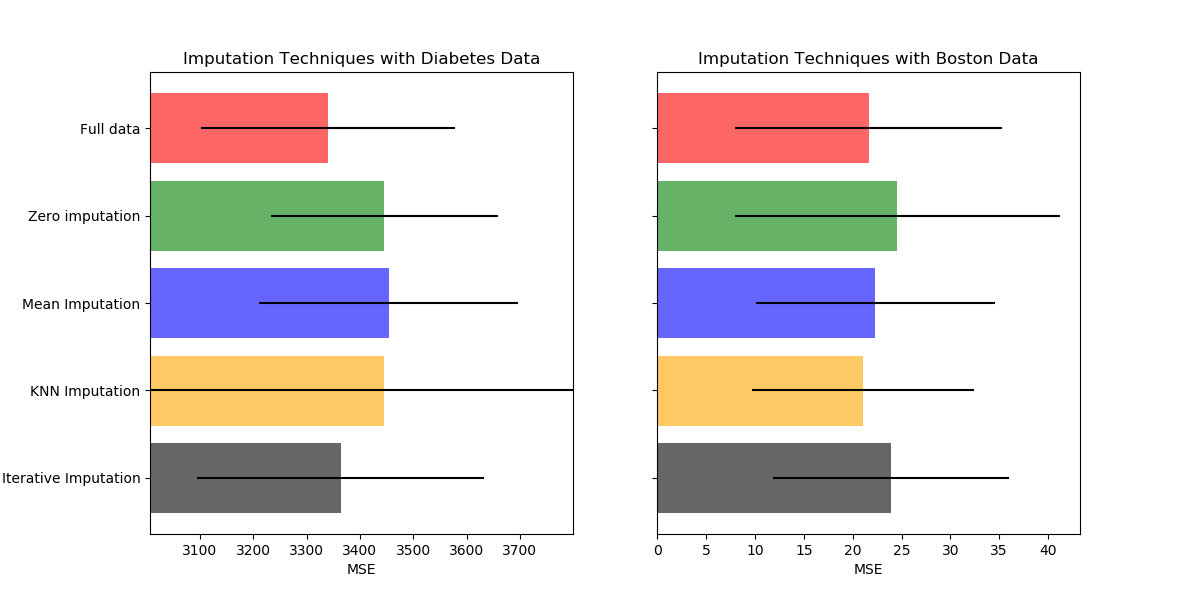# Imputing missing values before building an estimator¶

Missing values can be replaced by the mean, the median or the most frequent value using the basic sklearn.impute.SimpleImputer. The median is a more robust estimator for data with high magnitude variables which could dominate results (otherwise known as a ‘long tail’).

With KNNImputer, missing values can be imputed using the weighted or unweighted mean of the desired number of nearest neighbors.

Another option is the sklearn.impute.IterativeImputer. This uses round-robin linear regression, treating every variable as an output in turn. The version implemented assumes Gaussian (output) variables. If your features are obviously non-Normal, consider transforming them to look more Normal so as to potentially improve performance.

In addition of using an imputing method, we can also keep an indication of the missing information using sklearn.impute.MissingIndicator which might carry some information.print(__doc__)

import numpy as np
import matplotlib.pyplot as plt

# To use the experimental IterativeImputer, we need to explicitly ask for it:
from sklearn.experimental import enable_iterative_imputer  # noqa
from sklearn.ensemble import RandomForestRegressor
from sklearn.pipeline import make_pipeline, make_union
from sklearn.impute import (
SimpleImputer, KNNImputer, IterativeImputer, MissingIndicator)
from sklearn.model_selection import cross_val_score

rng = np.random.RandomState(0)

N_SPLITS = 5
REGRESSOR = RandomForestRegressor(random_state=0)

def get_scores_for_imputer(imputer, X_missing, y_missing):
estimator = make_pipeline(
make_union(imputer, MissingIndicator(missing_values=0)),
REGRESSOR)
impute_scores = cross_val_score(estimator, X_missing, y_missing,
scoring='neg_mean_squared_error',
cv=N_SPLITS)
return impute_scores

def get_results(dataset):
X_full, y_full = dataset.data, dataset.target
n_samples = X_full.shape
n_features = X_full.shape

# Estimate the score on the entire dataset, with no missing values
full_scores = cross_val_score(REGRESSOR, X_full, y_full,
scoring='neg_mean_squared_error',
cv=N_SPLITS)

# Add missing values in 75% of the lines
missing_rate = 0.75
n_missing_samples = int(np.floor(n_samples * missing_rate))
missing_samples = np.hstack((np.zeros(n_samples - n_missing_samples,
dtype=np.bool),
np.ones(n_missing_samples,
dtype=np.bool)))
rng.shuffle(missing_samples)
missing_features = rng.randint(0, n_features, n_missing_samples)
X_missing = X_full.copy()
X_missing[np.where(missing_samples), missing_features] = 0
y_missing = y_full.copy()

# Estimate the score after replacing missing values by 0
imputer = SimpleImputer(missing_values=0,
strategy='constant',
fill_value=0)
zero_impute_scores = get_scores_for_imputer(imputer, X_missing, y_missing)

# Estimate the score after imputation (mean strategy) of the missing values
imputer = SimpleImputer(missing_values=0, strategy="mean")
mean_impute_scores = get_scores_for_imputer(imputer, X_missing, y_missing)

# Estimate the score after kNN-imputation of the missing values
imputer = KNNImputer(missing_values=0)
knn_impute_scores = get_scores_for_imputer(imputer, X_missing, y_missing)

# Estimate the score after iterative imputation of the missing values
imputer = IterativeImputer(missing_values=0,
random_state=0,
n_nearest_features=5,
sample_posterior=True)
iterative_impute_scores = get_scores_for_imputer(imputer,
X_missing,
y_missing)

return ((full_scores.mean(), full_scores.std()),
(zero_impute_scores.mean(), zero_impute_scores.std()),
(mean_impute_scores.mean(), mean_impute_scores.std()),
(knn_impute_scores.mean(), knn_impute_scores.std()),
(iterative_impute_scores.mean(), iterative_impute_scores.std()))

mses_diabetes = results_diabetes[:, 0] * -1
stds_diabetes = results_diabetes[:, 1]

mses_boston = results_boston[:, 0] * -1
stds_boston = results_boston[:, 1]

n_bars = len(mses_diabetes)
xval = np.arange(n_bars)

x_labels = ['Full data',
'Zero imputation',
'Mean Imputation',
'KNN Imputation',
'Iterative Imputation']
colors = ['r', 'g', 'b', 'orange', 'black']

# plot diabetes results
plt.figure(figsize=(12, 6))
ax1 = plt.subplot(121)
for j in xval:
ax1.barh(j, mses_diabetes[j], xerr=stds_diabetes[j],
color=colors[j], alpha=0.6, align='center')

ax1.set_title('Imputation Techniques with Diabetes Data')
ax1.set_xlim(left=np.min(mses_diabetes) * 0.9,
right=np.max(mses_diabetes) * 1.1)
ax1.set_yticks(xval)
ax1.set_xlabel('MSE')
ax1.invert_yaxis()
ax1.set_yticklabels(x_labels)

# plot boston results
ax2 = plt.subplot(122)
for j in xval:
ax2.barh(j, mses_boston[j], xerr=stds_boston[j],
color=colors[j], alpha=0.6, align='center')

ax2.set_title('Imputation Techniques with Boston Data')
ax2.set_yticks(xval)
ax2.set_xlabel('MSE')
ax2.invert_yaxis()
ax2.set_yticklabels([''] * n_bars)

plt.show()


Total running time of the script: ( 0 minutes 16.465 seconds)

Estimated memory usage: 8 MB

Gallery generated by Sphinx-Gallery